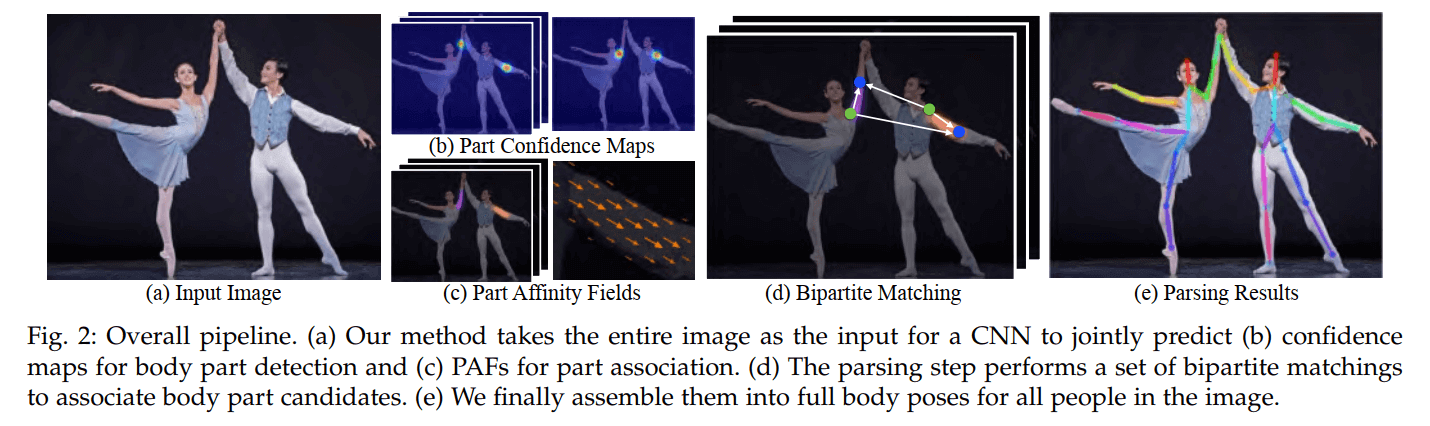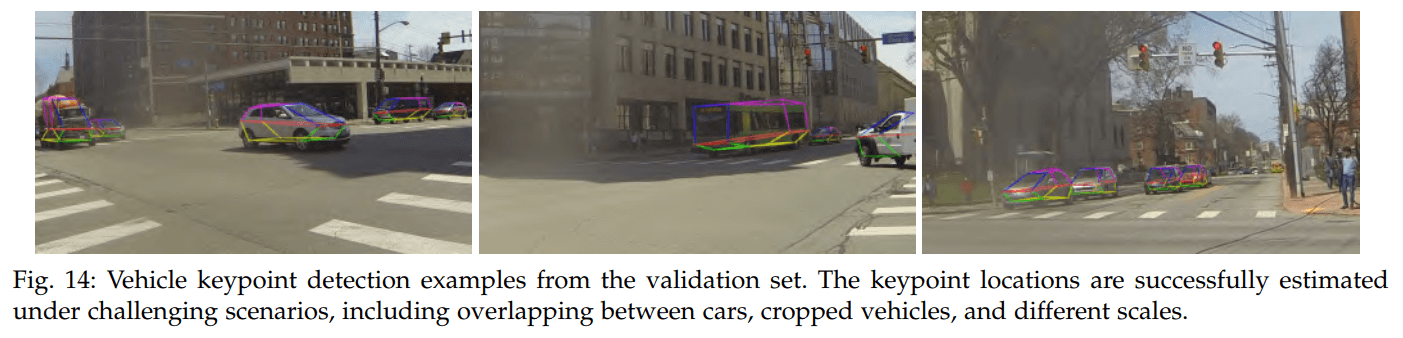# [CVPR 2017] OpenPose: Realtime Multi-Person 2D Pose Estimation using Part Affinity Fields

An efficient method for Realtime Multi-Person 2D Pose Estimation with an excellent implementation

Today’s topic is a paper named “Realtime Multi-Person 2D Pose Estimation using Part Affinity Fields” from CVPR 2017. This work has extraordinary contributions to the computer vision community because:

• It provides a realtime method for Multi-Person 2D Pose Estimation based on its bottom-up approach instead of detection-based approach in other works.
• The author opensources and also extends their work into the first real-time multi-person system to jointly detect human body, hand, facial, and foot keypoints (in total 135 keypoints) on single images - OpenPose. This library is being widely used today for various research works and production applications.

Some highlights of the paper:

• The first bottom-up presentation of association scores via Part Affinity Fields (PAFs).
• Invariant running time to the number of people in the image.
• Refined network increases both speed and accuracy by 200% and 7% respectively (2018 journal version)
• Can be generalized to any keypoint association task such as vehicle keypoint detection.

# IntroductionHuman Pose Estimation is a core problem for understanding of people in images and videos. In Single Person Pose Estimation, the problem is simplified by assuming the image has only one person. Multi Person Pose Estimation is a more difficult because there are multiple people in an image. Over years, there are many works focused on solving this problem.

A common approach is to follow a two-step framework which uses a human detector and solve the pose estimation for each human. This approach running time tends to grow with the number of people in the image and make the realtime performance a challenge.

In this paper, the author provides an bottom-up approach where the body parts are detected by the model and a final parsing is used to extract the pose estimation results. This approach can decouple the running time complexity from the number of people in the image.

The author also open-sources their work at OpenPose repository. This OpenPose system provides easy-to-use pipelines with command-line interfaces, Python API, Unity plugin. The system supports NVIDIA GPUs (CUDA), AMD GPUS (OPENCL) and CPU-only. They even provide portable executable binaries which can be simply download and use on Windows.

Here are some analyzed videos by the community:

# ArchitectureOverall Pipeline of the OpenPose architecture. (Source) The above figure is the overall pipeline of OpenPose. There are a few steps:

• First, the image is passed through a baseline network to extract feature maps. In the paper, the author uses the first 10 layers of VGG-19 model.
• Then, the feature maps are processed with multiple stages CNN to generate: 1) a set of Part Confidence Maps and 2) a set of Part Affinity Fields (PAFs)
• Part Confidence Maps: a set of 2D confidence maps S for body part locations. Each joint location has a map.
• Part Affinity Fields (PAFs): a set of 2D vector fields L which encodes the degree of association between parts.
• Finally, the Confidence Maps and Part Affinity Fields are processed by a greedy algorithm to obtain the poses for each person in the image.

# Confidence Maps

A Confidence Map is a 2D representation of the belief that a particular body part can be located in any given pixel.

Let $J$ be the number of body part locations (joints). Then, Confidence Maps are the set $S = (S_1, S_2, .., S_J)$ where $S_j \in R^{w \times h}, j \in { 1…J }$.

In summary, each map is correspond for a joint and has the same size as the input image.

# Part Affinity Fields (PAFs)

A Part Affinity Field (PAF) is a set of flow fields that encodes unstructured pairwise relationships between body parts.

Each pair of body parts has a (PAF), i.e neck, nose, elbow, etc,.

Let $C$ be the number of pairs of body parts. Then, Part Affinity Fields (PAFs) are the set $L = (L_1, L_2, …, L_C)$ where $L_c \in R^{w \times h \times 2}, c \in {1…C}$.

If a pixel is on a limb (body part), the value in $L_c$ at that pixel is a 2D unit vector from the start joint to the end joint.

# Multi-stage CNNThis is the architecture of the multi-stage CNN from the 2018 journal version of the OpenPose. There are a few steps as following:

• Stage 1: Compute the part affinity fields (PAFs), $L^1$ from the feature maps of the base network, $F$. Let $\phi^1$ be the CNN at the stage 1. $$L^1 = \phi^1(F)$$
• Stage $t$ to Stage $T_P$: refines the predictions of PAFs from previous stage using the feature maps $F$ and the previous PAFs $L^{t-1}$. Let $\phi^t$ be the CNN at the stage t. $$L^t = \phi^t(F, L^{t-1}), \forall 2 \leq t \leq T_P$$
• After $T_P$ iterations, the process is repeated for the confidence maps detection, starting in the most updated PAF prediction. Let $\rho^t$ be the CNN at the stage t. The process is repeated for $T_C$ iteration. $$S^{T_P} = \rho^t(F, L^{T_P}), \forall t = T_P$$ $$S^t = \rho^t(F, L^{T_P}, S^{t-1}), \forall T_P < t \leq T_P + T_C$$
• The final $S$ and $L$ are the confidence maps and the part affinity fields (PAFs) that will be further processed by the greedy algorithm.

Note:

• This multi-stage CNN is from the 2018 journal version. In the original CVPR 2017 version, they refined both the confidence maps and the part affinity fields (PAFs) at each stage. Therefore, they required much more computation and time at each stage. In the new approach, the author finds that the new approach increases both speed and accuracy by 200% and 7% respectively.

# Multi-Person Parsing using PAFs

In this section, we will give you an overview of the greedy algorithm which is used to parse poses of multiple people from confidence maps and part affinity fields.

Many other blog posts show how difficult this problem is. However, there aren’t many detailed explanations of this steps.

Fortunately, we found an excellent implementation of this paper by tensorboy which has detailed documentations and straightforward code. We suggest that you check the repository out and try it yourself.

The parsing process can be summarized into three steps:

• Step 1: Find all joints locations using the confidence maps.
• Step 2: Find which joints go together to form limbs (body parts) using the part affinity fields and joints in step 1.
• Step 3: Associate limbs that belong to the same person and get the final list of human poses.

## Step 1: Find all joints locations using the confidence maps.

Inputs:

• Confidence maps, $S = (S_1, S_2, .., S_J)$ where $S_j \in R^{w \times h}, j \in { 1…J }$
• Up-sampling scale: the different in width/height of input image and the confidence maps.

Outputs:

• joints_list: a list of joint locations of size $J$ where each item is a list of peaks (x, y, probability).
• For example, joints_list length is 18 for 18 joint locations (nose, neck, etc.) and items in joints_list are lists of different lengths which stores the peak information (x, y location and probability score) for each joint location.

Process:

• For each joint from 1 to J:
• Get the corresponding 2D heatmap for the joint in confidence maps.
• Find the peaks by thresholding the 2D heatmap.
• For each peak:
• Take a patch around the peak in the heap
• Scale up the patch using the up-sampling scale.
• Get the maximum peak location in the scaled up patch.
• Add the peak information to the list peaks of the joint

## Step 2: Find which joints go together to form limbs (body parts) using the part affinity fields and joints in step 1.

Inputs:

• joints_list: the output of step 1.
• Part affinity fields (PAFs): $L = (L_1, L_2, …, L_C)$ where $L_c \in R^{w \times h \times 2}, c \in {1…C}$.
• Up-sampling scale: the different in width/height of input image and the PAFs maps.
• Number of intermediate points: the number of intermediate points between a source and destination joints to get the PAFs value. Outputs:

• connected_limbs: a list of connected limbs of size $C$ where each item is a list of all limbs of that type found.

• Each limb information contains: id of source joint, id of target joint and a score of how good the connection is.

Process:

• Scale up the PAFs to the input size using the up-sampling scale
• For each limb type, i.e left wrist_elbow:
• Get all source joint peaks and destination joint peaks, i.e all left wrist peaks and all left elbow peaks.
• If length of either source or destination peaks is 0, then skip this limb.
• Create a list to store all limb connection candidates.
• For each source peak and each target peak:
1. Get the direction vector by subtracting destination and source locations
2. Normalize the direction vector to a unit vector
3. Get PAFs values at each intermediate points between the source and destination peaks.
4. Calculate the score of the current limb connection by averaging the PAFs values.
5. Add a score to penalize the long distance limb: min(0.5 * paf_height / limb_dist - 1, 0)
6. Add the current limb connections to the limb connection candidates
• Sort the limb connection candidates
• For each connection candidates:
• Add the connection to the final list if the source and destination is not selected for any connection.

## Step 3: Associate limbs that belong to the same person and get the final list of human poses.

Inputs:

• joints_list: from step 1
• connected_limbs: from step 2

Outputs:

• poses: a list of human poses for each person in the image. Each item contains the joint locations for that person.

Process:

• For each limb types and for each connection in connected_limbs of that type:
• Find the persons that associated with either joint of the current connection
• If there is no person: Create a new person with the current connection
• If there is 01 person: Add the current connection to that person
• If there is 02 person: Merge these 02 persons into 01 person.
• Remove any person with very few joints.

# Vehicle Pose Estimation

In the 2018 journal version, the author demonstrates that this approach can be applied to any keypoint annotation task. The following image is the result on a vehicle keypoint dataset:# Implementations

• The best implementation of OpenPose is from the OpenPose repository which is implemented C++. This OpenPose system provides easy-to-use pipelines with command-line interfaces, Python API, Unity plugin. The system supports NVIDIA GPUs (CUDA), AMD GPUS (OPENCL) and CPU-only. They even provide portable executable binaries which can be simply download and use on Windows.
• An excellent PyTorch implementation of this paper by tensorboy which has detailed documentations and straightforward code.
• A Tensorflow implementation with multiple base network is provided by ildoonet.